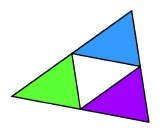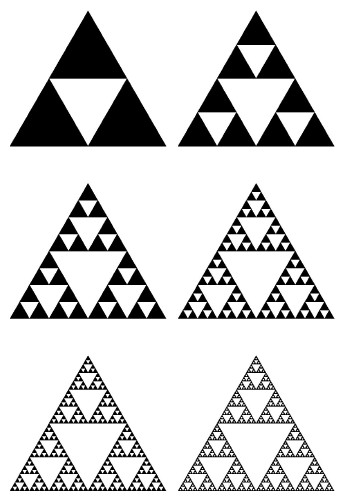Scaling and Growth ExponentsDecomposing a triangle into four congruent triangles.

When we double the size of an object, the lengths of edges double, the area of square faces goes up by four, and the volume of cubical pieces goes up by a factor of eight. In general we expect that a quantity will increase by a power of two when we double the size, and this growth exponent is the same as the dimension of the quantity. But what of exponents that are not integers? Surprisingly, there are quantities that go up by a power between two and four when their size is doubled. There has been a great interest in recent years in the beautiful images that come from "fractal" objects with noninteger growth exponents.

One of the most popular examples of this phenomenon was invented by the Polish mathematician Waclaw Sierpinski. Any triangle can be cut into four congruent triangles, and the first step in creating Sierpinski's figure is to remove the middle triangle. The next step is to remove the middle of each of the remaining triangles. Repeating this over and over again creates what is known as the "Sierpinski gasket."Six steps in the creation of the Sierpinski gasket.

This object has the remarkable property that doubling its size produces a figure composed of three copies of the original figure. If we double the size of something of dimension one, we get two copies of the original; if we double the size of something of dimension two, we get four copies of the original. The Sierpinski gasket has a dimension such that when we raise two to that exponent, we get three. There is no whole number with this property, and in fact the dimension of the Sierpinski gasket lies somewhere between one and two. Specifically, it is the logarithm of three to the base two.

Another figure that is created by such an infinite process is the Koch snowflake. The snowflake starts with an equilateral triangle. On each of the 3 edges of that triangle, we erect a triangle one-third as large. We then erect a triangle one-ninth as large as the original on each of the 12 edges of the previous figure. Each of these edges is thus replaced by four smaller edges one-third as long as the original. It follows that the total perimeter is multiplied by 4/3 at each stage. Take this process to its limit and we have Koch's snowflake. (In the illustration, we expand the size of the figure at each stage.)Five steps in the creation of the Koch snowflake.